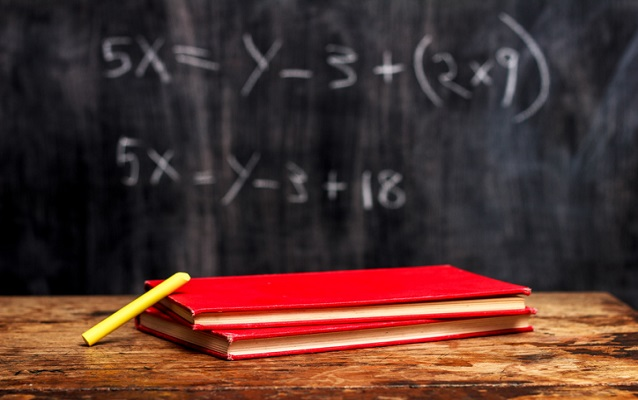# CAT Preparation 2022: Quantitative Aptitude (QA) Solved Questions

5 mins read26.2K Views Comment
clickHere
Updated on Jul 4, 2022 13:50 IST

Busy with CAT preparation 2022? Shiksha.com brings to you solved problems on QA for practice. Try them and check your QA preparation level for CAT 2022.Quantitative Aptitude (QA) is common to all MBA entrance exams. One can say that it is one of the major skills that is tested for selection of an MBA candidate. QA covers major topics of Mathematics studied in Class 9 and 10 but of advance level. Geometry, Algebra, Trigonometry, Mensuration and Modern Math form the basis of QA section.

QA preparation for CAT and other MBA entrance exams such as XAT, SNAP, CMAT, NMAT, MAT and IIFT exam, should be divided into two parts.

1. Understanding topics and concepts
2. Practicing sample questions and mock tests

CAT Maths questions are calculation intensive and even if you have studied it till graduation, you need to have complete clarity of concepts and theorems. Once there is complete conceptual clarity, start solving sample questions to improve efficiency in application of formulae for 100 per cent accuracy in solving. CAT algebra questions and CAT geometry questions are usually of high difficulty level and require practice.

Given below are a few sample questions of CAT Quantitative Aptitude with solutions. Attempt these questions and check your preparation level for CAT exam and other MBA entrance exams.

## CAT Preparation 2022: Solved CAT Quantitative Aptitude Questions for Practice

CAT Percentage Question:

DIRECTIONS for questions 1 to 5: Answer the question independently.

1. A dishonest shopkeeper sells sugar at cost price but makes 25% profit by using faulty weights. He decides to give a discount to attract more customers. What percent of discount should he offer to make 12% profit?

1] 15.2%                2] 12.5%                3] 10.4%                4] 13%

2. N is a natural number having the sum of it’s digits as 3. If 101314, then how many values can ‘N’ assume?

1] 93       2] 91       3] 78       4] 105

3. Two barrels A and C contain alcohol and water in the ratio 1:a and 1:c, respectively. Equal quantity of mixtures from barrels A and C are mixed to get a mixture with alcohol and water in the ratio 1:b, where b is the arithmetic mean of a and c. What can be the value of a + b + c, if a, b and c are integers?

1] 14       2] 10       3] 15       4] 8

4. Given 2 positive integers, ‘x’ and ‘y’ with x

1] 10 and 1990    2] 900 and 1880    3] 1 and 1890    4] 1 and 900

5. Ajay plans to host a lunch for his friends at a restaurant. He decides to keep 1 starter and 2 main courses for the lunch. From the menu, he realizes that there are only 15 ways to fulfill his requirement. What can be the total number of dishes available on the menu?

1] 4         2] 5         3] 6         4] 7

6. If ‘a’ is positive and a4 – 62a2   + 1 = 0, find the value of a3 + 1/a3

1] 648    2] 392    3] 488    4] 512

7. A six-faced unbiased die is thrown ten times. The results of these ten throws are written side by side, from left to right so as to form a ten-digit number, such that the first four digits form a four digit number which is the greatest four-digit square possible. Similarly, the next three digits, next two digits and the last digit form the greatest possible three-digit, two-digit and one-digit perfect squares respectively. Find the sum of digits of that ten-digit number.

1] 45       2] 46       3] 42       4] Cannot be determined

8. On a 120 kms racing track, if P and Q start driving in the same direction from the same point and at the same time, then P wins the race by 25 minutes. If they drive towards each other from the opposite ends on the same track starting at the same time, the distances that P and Q cover when they meet are in the ratio 3:2. Find the speed of P’s car.

1] 96 kmph            2] 48 kmph            3] 144 kmph         4] 72 kmph

9. PQR is an isosceles right angled triangle with ?QPR = 90°. Semicircles are drawn with PQ and PR as diameters. Find the ratio of the area of the shaded region to the area of the triangle PQR.

11. A task was given to a manager who had 14 members in his team. At any moment of time exactly 10 members worked simultaneously on the task. Since, the manager had to be fair in his work allocation, he allocated the task among the workers in such a way that each member worked on the task for exactly ‘t’ minutes. If the task was completed in 210 minutes, then find the value of ‘t’. (Assume the efficiency of each of the members of the team is the same)

1] 15       2] 150    3] 21       4] 60

CHECK OUT:

Content courtesy:

Alok Bansal has been the Director (2012-2018) of a popular coaching institute that trains students for MBA entrance exams like CAT, XAT  and GMAT.

Try this CAT Mock Test, Powered by IMS, to assess your preparation level and time-management skill.

Free CAT Mock Test 1

Try second CAT Mock Test, Powered by IMS, to assess your preparation level and time-management skill.

Free CAT Mock Test 2

clickHere

This account contains a repository of informative articles by external authors with domain expertise in various aspects of guiding students on how to go about pursuing their undergraduate and postgraduate studies in... Read Full Bio